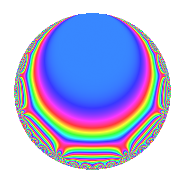# Properties

 Label 1344.2.s.bLevel 1344 Weight 2 Character orbit 1344.s Analytic conductor 10.732 Analytic rank 0 Dimension 4 CM No Inner twists 2

# Related objects

## Newspace parameters

 Level: $$N$$ = $$1344 = 2^{6} \cdot 3 \cdot 7$$ Weight: $$k$$ = $$2$$ Character orbit: $$[\chi]$$ = 1344.s (of order $$4$$ and degree $$2$$)

## Newform invariants

 Self dual: No Analytic conductor: $$10.7318940317$$ Analytic rank: $$0$$ Dimension: $$4$$ Relative dimension: $$2$$ over $$\Q(i)$$ Coefficient field: $$\Q(\zeta_{8})$$ Coefficient ring: $$\Z[a_1, \ldots, a_{11}]$$ Coefficient ring index: $$2$$ Sato-Tate group: $\mathrm{SU}(2)[C_{4}]$

## $q$-expansion

Coefficients of the $$q$$-expansion are expressed in terms of a basis $$1,\beta_1,\beta_2,\beta_3$$ for the coefficient ring described below. We also show the integral $$q$$-expansion of the trace form.

 $$f(q)$$ $$=$$ $$q + ( 1 - \beta_{2} ) q^{3} + ( -1 - \beta_{1} + \beta_{2} + \beta_{3} ) q^{5} + q^{7} + ( -1 - 2 \beta_{2} ) q^{9} +O(q^{10})$$ $$q + ( 1 - \beta_{2} ) q^{3} + ( -1 - \beta_{1} + \beta_{2} + \beta_{3} ) q^{5} + q^{7} + ( -1 - 2 \beta_{2} ) q^{9} + ( 1 - \beta_{1} - 2 \beta_{2} + 2 \beta_{3} ) q^{11} + ( -3 - 3 \beta_{1} - \beta_{2} - \beta_{3} ) q^{13} + ( 1 - 3 \beta_{1} + 2 \beta_{2} ) q^{15} + ( 4 \beta_{1} - 2 \beta_{2} ) q^{17} + ( 1 - \beta_{1} + \beta_{2} - \beta_{3} ) q^{19} + ( 1 - \beta_{2} ) q^{21} + ( 2 \beta_{1} + 4 \beta_{2} ) q^{23} + ( \beta_{1} - 4 \beta_{2} ) q^{25} + ( -5 - \beta_{2} ) q^{27} + ( -3 + 3 \beta_{1} ) q^{29} + ( 2 \beta_{1} - 6 \beta_{2} ) q^{31} + ( -3 - 5 \beta_{1} - 3 \beta_{2} + \beta_{3} ) q^{33} + ( -1 - \beta_{1} + \beta_{2} + \beta_{3} ) q^{35} + ( -1 + \beta_{1} - 2 \beta_{2} + 2 \beta_{3} ) q^{37} + ( -5 - \beta_{1} + 2 \beta_{2} - 4 \beta_{3} ) q^{39} + ( -6 + 4 \beta_{3} ) q^{41} + ( 1 + \beta_{1} + 2 \beta_{2} + 2 \beta_{3} ) q^{43} + ( 5 - 3 \beta_{1} + \beta_{2} - 3 \beta_{3} ) q^{45} + 4 \beta_{3} q^{47} + q^{49} + ( -4 + 4 \beta_{1} - 2 \beta_{2} + 4 \beta_{3} ) q^{51} + ( -3 - 3 \beta_{1} - 2 \beta_{2} - 2 \beta_{3} ) q^{53} + ( 6 - 2 \beta_{3} ) q^{55} + ( 3 + \beta_{1} - 2 \beta_{3} ) q^{57} + ( 3 - 3 \beta_{1} - 5 \beta_{2} + 5 \beta_{3} ) q^{59} + ( 1 + \beta_{1} + \beta_{2} + \beta_{3} ) q^{61} + ( -1 - 2 \beta_{2} ) q^{63} + ( 2 \beta_{1} - 4 \beta_{2} ) q^{65} + ( 7 - 7 \beta_{1} ) q^{67} + ( 8 + 2 \beta_{1} + 4 \beta_{2} + 2 \beta_{3} ) q^{69} + 6 \beta_{1} q^{71} + ( 8 \beta_{1} - 2 \beta_{2} ) q^{73} + ( -8 + \beta_{1} - 4 \beta_{2} + \beta_{3} ) q^{75} + ( 1 - \beta_{1} - 2 \beta_{2} + 2 \beta_{3} ) q^{77} -2 \beta_{1} q^{79} + ( -7 + 4 \beta_{2} ) q^{81} + ( 1 + \beta_{1} - 7 \beta_{2} - 7 \beta_{3} ) q^{83} + ( 8 - 8 \beta_{1} + 6 \beta_{2} - 6 \beta_{3} ) q^{85} + ( -3 + 3 \beta_{1} + 3 \beta_{2} + 3 \beta_{3} ) q^{87} + ( -10 - 4 \beta_{3} ) q^{89} + ( -3 - 3 \beta_{1} - \beta_{2} - \beta_{3} ) q^{91} + ( -12 + 2 \beta_{1} - 6 \beta_{2} + 2 \beta_{3} ) q^{93} + ( -6 + 4 \beta_{3} ) q^{95} -2 q^{97} + ( -9 - 7 \beta_{1} - 4 \beta_{3} ) q^{99} +O(q^{100})$$ $$\operatorname{Tr}(f)(q)$$ $$=$$ $$4q + 4q^{3} - 4q^{5} + 4q^{7} - 4q^{9} + O(q^{10})$$ $$4q + 4q^{3} - 4q^{5} + 4q^{7} - 4q^{9} + 4q^{11} - 12q^{13} + 4q^{15} + 4q^{19} + 4q^{21} - 20q^{27} - 12q^{29} - 12q^{33} - 4q^{35} - 4q^{37} - 20q^{39} - 24q^{41} + 4q^{43} + 20q^{45} + 4q^{49} - 16q^{51} - 12q^{53} + 24q^{55} + 12q^{57} + 12q^{59} + 4q^{61} - 4q^{63} + 28q^{67} + 32q^{69} - 32q^{75} + 4q^{77} - 28q^{81} + 4q^{83} + 32q^{85} - 12q^{87} - 40q^{89} - 12q^{91} - 48q^{93} - 24q^{95} - 8q^{97} - 36q^{99} + O(q^{100})$$

Basis of coefficient ring:

 $$\beta_{0}$$ $$=$$ $$1$$ $$\beta_{1}$$ $$=$$ $$\zeta_{8}^{2}$$ $$\beta_{2}$$ $$=$$ $$\zeta_{8}^{3} + \zeta_{8}$$ $$\beta_{3}$$ $$=$$ $$-\zeta_{8}^{3} + \zeta_{8}$$
 $$1$$ $$=$$ $$\beta_0$$ $$\zeta_{8}$$ $$=$$ $$($$$$\beta_{3} + \beta_{2}$$$$)/2$$ $$\zeta_{8}^{2}$$ $$=$$ $$\beta_{1}$$ $$\zeta_{8}^{3}$$ $$=$$ $$($$$$-\beta_{3} + \beta_{2}$$$$)/2$$

## Character Values

We give the values of $$\chi$$ on generators for $$\left(\mathbb{Z}/1344\mathbb{Z}\right)^\times$$.

 $$n$$ $$127$$ $$449$$ $$577$$ $$1093$$ $$\chi(n)$$ $$-1$$ $$-1$$ $$1$$ $$-\beta_{1}$$

## Embeddings

For each embedding $$\iota_m$$ of the coefficient field, the values $$\iota_m(a_n)$$ are shown below.

For more information on an embedded modular form you can click on its label.

Label $$\iota_m(\nu)$$ $$a_{2}$$ $$a_{3}$$ $$a_{4}$$ $$a_{5}$$ $$a_{6}$$ $$a_{7}$$ $$a_{8}$$ $$a_{9}$$ $$a_{10}$$
239.1
 0.707107 + 0.707107i −0.707107 − 0.707107i −0.707107 + 0.707107i 0.707107 − 0.707107i
0 1.00000 1.41421i 0 0.414214 + 0.414214i 0 1.00000 0 −1.00000 2.82843i 0
239.2 0 1.00000 + 1.41421i 0 −2.41421 2.41421i 0 1.00000 0 −1.00000 + 2.82843i 0
911.1 0 1.00000 1.41421i 0 −2.41421 + 2.41421i 0 1.00000 0 −1.00000 2.82843i 0
911.2 0 1.00000 + 1.41421i 0 0.414214 0.414214i 0 1.00000 0 −1.00000 + 2.82843i 0
 $$n$$: e.g. 2-40 or 990-1000 Significant digits: Format: Complex embeddings Normalized embeddings Satake parameters Satake angles

## Inner twists

Char. orbit Parity Mult. Self Twist Proved
1.a Even 1 trivial yes
48.k Even 1 yes

## Hecke kernels

This newform can be constructed as the kernel of the linear operator $$T_{5}^{4} + 4 T_{5}^{3} + 8 T_{5}^{2} - 8 T_{5} + 4$$ acting on $$S_{2}^{\mathrm{new}}(1344, [\chi])$$.